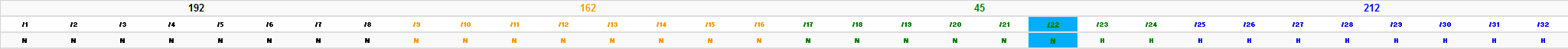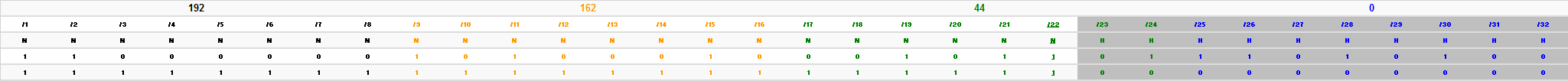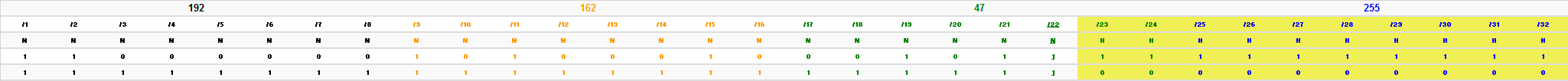I can follow the “normal” /30 method, using borrowed bits from the fourth octet, but I find it difficult to figure out what happens when you are using the third, or possibly the second, octet.

If I may pose an example with a classless address:

``````IP address = 192.162.45.212 /22
Therefore S/M = 255.255.252.0
``````

I believe that this would be demonstrated as : N.N.nnnnnnhh.H

Using the borrowing principal, what is the Network address, and then doing an “Anding” calculation I come up with the following:

``````11000000—10100010—00101101—11010100 = IP address
11111111—11111111—11111100—00000000 = S/M

1st Host = 192.162.44.1
``````

What I am puzzled about is how do you calculate the last host, and the broadcast? Although based on your explanation, the last host will be one address lower than the broadcast. My problem is that I can figure out what you do with the two “hh” bits that are in octet 3.

First of all I just wanted to thank the author for their detailed e-mail. It gives me a much better understanding of which areas I need to focus on in my response. This in turn ensures that I’m providing them with the information they need as opposed to rambling on about something they already know :)

Let’s begin by breaking down the author’s e-mail. His example IP address is: 192.162.45.212 /22 so let’s find out where exactly /22 sits.Click to enlarge

As highlighted above, we can see that /22 resides in the third octet. This tells us that we have two host bits left over in the third octet and eight host bits in the fourth octet.

Let’s now do as the author did figure out what the network address is by AND’ing the IP address and the subnet mask.Click to enlargeClick to enlarge

Next let’s look at the fourth octet. As all 8 bits are “Host” bits we’ll need to flip them all to 1’s and again add them all together, like so: 128 + 64 + 32 + 16 + 8 + 4 + 2 + 1 = 255.The first two host bits we see are /23 and /24 and are the bits which the author referred to when he said “My problem is that I can figure out what you do with the two “hh” bits that are in octet 3.”. As these are the two least significant bits in this octet we know that their values are 2 and 1 respectively. Therefore by switching these “Host” bits to 1 and adding them together we get a total of 3. Then, when we add this 3 to the network address’ third octet of 44, we get a total of 47. That’s all there is to it! :)

When we put these two pieces of information together we get the broadcast address 192.162.47.255. By subtracting one from this address we then get the last usable address 192.162.47.254.

And there you have it! All we had to do was flip all host bits to 1 and then added the results to the network address’ octets and we found the broadcast address. We then subtracted one from this address and we found the last usable address. For completeness I’ll run the information we have collected through my Subnetting Made Easy Formula:

See the Subnetting Made Easy - Index post for links to all of the posts in this series.

As always, if you have any questions or have a topic that you would like me to discuss, please feel free to post a comment at the bottom of this blog entry, e-mail at will@oznetnerd.com, or drop me a message on Reddit (OzNetNerd).

Note: The opinions expressed in this blog are my own and not those of my employer.

Tags:

Categories:

Updated: